## Saturday, 23 December 2017

### How to find log (alpha+ i beta), Where alpha and beta are real

Here is the video to show the details of solving this problem. It is an important problem for basic understanding about the logarithm of real numbers. It is also important for understand about the logarithm of complex numbers and complex roots.  Please see this video and ask questions if you have any. For many other videos of mathematics problem please subscribe my YouTube channel.

Hope you will enjoy and take proper participate in my YouTube channel.

## Tuesday, 24 October 2017

### How to Evaluate an Integral

In this video you will see how to evaluate an Integral. This video shows an example, by this example you will see about how to evaluate an Integral. If you have any question or want solution of any mathematical problem of related topic, please write on the comment box. May be the next video is solution process of your mathematical problem.

## Thursday, 21 September 2017

### Introduction of Circle

All of my lessons and teaching videos are in English and most of them are for students of Logistics Management. But many of mys students and followers are always asking for lessons and teaching videos in Hindi. Now I have posted my first video lesson of Mathematics in Hindi. here is my Video.

## Friday, 10 October 2014

### Calculation Of Specific Gravity

Calculation Of Specific Gravity:-
The Specific Gravity (it is also written as sp.gr.) of a substance is the ratio of the weight of a given volume of the substance to the weight of an equal volume of some standard substance (which is taken as water at 4degreeC)

Obviously it is a pure number and processes no dimensions.

Hence the Specific gravity of a substance

=(the weight of volume  v   of the substance)/(the weight of volume   v  of the standard substance)

=(the weight of a unit volume of the substance)/(the weight of a unit volume of the standard substance)

=(the mass of a unit volume of the substance)/(the mass of a unit volume of the standard substance)     (as weight=mass*g)

=(the density of the substance)/(the density of standard substance)

This is the final step for Specific Gravity.

True specific gravity can be expressed mathematically as: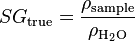$SG_\text{true} = \frac {\rho_\text{sample}}{\rho_{\rm H_2O}}$
where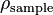$\rho_\text{sample}\,$ is the density of the sample and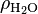$\rho_{\rm H_2O}$ is the density of water.
The apparent specific gravity is simply the ratio of the weights of equal volumes of sample and water in air: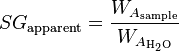$SG_\text{apparent} = \frac {W_{A_\text{sample}}}{W_{A_{\rm H_2O}}}$
where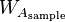$W_{A_\text{sample}}$ represents the weight of sample and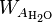$W_{A_{\rm H_2O}}$ the weight of water, both measured in air.
It can be shown that true specific gravity can be computed from different properties: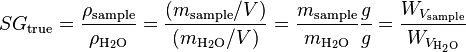$SG_\text{true} = \frac {\rho_\text{sample}}{\rho_{\rm H_2O}} = \frac {(m_\text{sample}/V)}{(m_{\rm H_2O}/V)} = \frac {m_\text{sample}}{m_{\rm H_2O}} \frac{g}{g} = \frac {W_{V_\text{sample}}}{W_{V_{\rm H_2O}}}$

where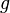$g$ is the local acceleration due to gravity,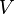$V$ is the volume of the sample and of water (the same for both),${\rho_\text{sample}}$ is the density of the sample,$\rho_{\rm H_2O}$ is the density of water and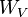$W_V$ represents a weight obtained in vacuum.

## Thursday, 25 September 2014

### Number Relation in table 9 for Primary Students

In mathematics, a multiplication table (sometimes, less formally, a times table) is a mathematical table used to define a multiplication operation for an algebraic system.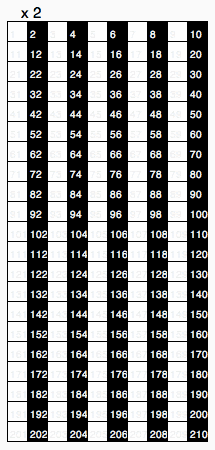Visual representation of the different multiplication tables from 2 to 50
The decimal multiplication table was traditionally taught as an essential part of elementary arithmetic around the world, as it lays the foundation for arithmetic operations with base-ten numbers. Many educators believe it is necessary to memorize the table up 9 multiply by 9.

Number Relation in table 9 :-

There is a relation between the digits of all numbers in Multiplication Table of 9

9*1=09   where   0+9=9
9*2=18    where   1+8=9
9*3=27    where   2+7=9
9*4=36    where   3+6=9
9*5=45    where   4+5=9
9*6=54    where   5+4=9
9*7=63     where   6+3=9
9*8=72     where   7+2=9
9*9=81     where   8+1=9
9*10=90   where   9+0=9

It proves that every number of multiplication table of 9 when added to make a single number then this number is also 9.

This very interesting and very Important for every primary students to know this number relation

## Monday, 22 September 2014

### Fluid PressurePressure at any point of a fluid. :-

Pressure is the amount of force acting per unit area. The symbol of pressure is p or P

Mathematically: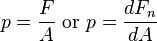$p = \frac{F}{A}\ \mbox{or}\ p = \frac{dF_n}{dA}$
where: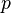$p$ is the pressure,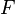$F$ is the normal force,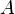$A$ is the area of the surface on contact.
Pressure is a scalar quantity. It relates the vector surface element (a vector normal to the surface) with the normal force acting on it. The pressure is the scalar proportionality constant that relates the two normal vectors: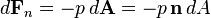$d\mathbf{F}_n=-p\,d\mathbf{A} = -p\,\mathbf{n}\,dA$

Uniform Pressure :-
The pressure on a Plane area is uniform when the thrust on any portion of it is proportional to the area of that portion, and the pressure is measured by the thrust on a unit area.

Mean Pressure :-
The mean pressure on a plane area is the uniform pressure on it which will give the same resultant thrust as the actual one.

Pressure at a Point :-
The Pressure at any point of an area is the limit of the mean pressure on an indefinitely small area enclosing the point.

## Our Latest Post

### How to find log (alpha+ i beta), Where alpha and beta are real

Here is the video to show the details of solving this problem. It is an important problem for basic understanding about the logarithm of re...